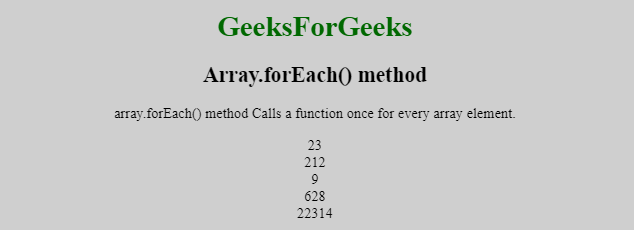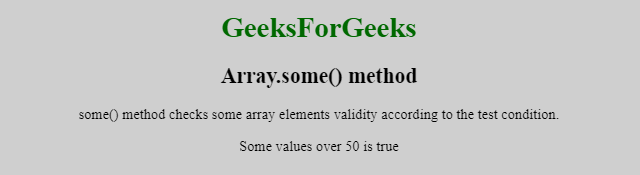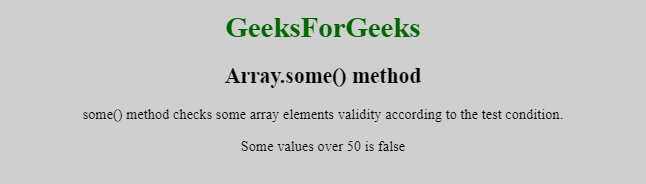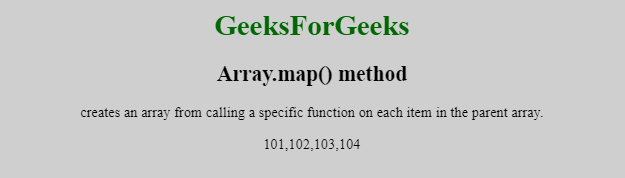GFG App
Open AppBrowser
Continue

# JavaScript | Array Iteration Methods

Array iteration methods perform some operation on each element of array. There are some different examples of Array iteration methods are given below.
Array.forEach() function: The array.forEach() function calls the provided function(a callback function) once for each element of the array. The provided function is user defined, it can perform any kind of operation on array.
Syntax:

```array.forEach(function callback(value, index, array){
}[ThisArgument]);```

Parameters: This function accepts three parameters as mentioned above and described below:

• value: This is the current value being processed by the function.
• index: Item index is the index of the current element which was being processed by the function.
• array: The array on which the .forEach() function was called.

Example: This example uses forEach method on array for iterating every element of array and printing every element of array in new line.

## html

 `` `<``html``>` `    `  `<``head``>` `    ``<``title``>` `        ``JavaScript Array.forEach() function` `    ``` ``   `<``body` `style = "text-align:center;"> `   `    ``<``h1` `style = "color:green;" > ` `        ``GeeksForGeeks ` `    ```   `    ``<``h2``>Array.forEach() method` `    `  `    ``<``p``>` `        ``array.forEach() method Calls a function` `        ``once for every array element.` `    ``` `    `  `    ``<``p` `id="GFG">` `    `  `    ``<``script``>` `        ``var emptytxt = "";` `        ``var No = [23, 212, 9, 628, 22314];` `        ``No.forEach(itrtFunction);` `        `  `        ``document.getElementById("GFG").innerHTML = emptytxt;` `        `  `        ``function itrtFunction(value, index, array) {` `            ``emptytxt = emptytxt + value + "<``br``>"; ` `        ``}` `    ``` ``   `                    `

Output:Array.some() function: The array.some() function check whether at least one of the elements of the array satisfies the condition checked by the argument function.
Syntax:

`array.some(arg_function(value, index, array), thisArg);`

Parameters: This function accepts three parameters as mentioned above and described below:

• value: This is the current value being processed by the function.
• index: Item index is the index of the current element which was being processed by the function.
• array: The array on which the .some() function was called.

Example: This example checks all value of array, if some value is greater than 50 then it return true and if all element of array is less than 18 then it return false.

## html

 `` `<``html``>` `    `  `<``head``>` `    ``<``title``>` `        ``JavaScript Array.some() function` `    ``` ``   `<``body` `style = "text-align:center;"> `   `    ``<``h1` `style = "color:green;" > ` `        ``GeeksForGeeks ` `    ```   `    ``<``h2``>Array.some() method` `    `  `    ``<``p``>` `        ``some() method checks some array elements ` `        ``validity according to the test condition.` `    ``` `    `  `    ``<``p` `id="GFG">` `    `  `    ``<``script``>` `        ``var numArray = [41, 2, 14, 29, 49];` `        ``var someOver50 = numArray.some(myFunction);` `        `  `        ``document.getElementById("GFG").innerHTML` `            ``= "Some values over 50 is " + someOver50;` `        `  `        ``function myFunction(value, index, array) {` `            ``return value > 50;` `        ``}` `    ``` ``   `                    `

Output:

• If any one value of array is greater than 50:• If all value of array is less than 50:Array.map() function: The array.map() function creates an array from calling a specific function on each item in the parent array and it does not change the value or element of array.
Syntax:

```array.map(function(value, index, array){
}[ThisArgument]);```

Parameters: This function accepts three parameters as mentioned above and described below:

• value: This is the current value being processed by the function.
• index: Item index is the index of the current element which was being processed by the function.
• array: The array on which the .map() function was called.

Example: This example perform sum operation on every element of array and display output. It does not change the values of original array.

## html

 `` `<``html``>`   `<``head``>` `    ``<``title``>` `        ``JavaScript Array.map() function` `    ``` ``   `<``body` `style = "text-align:center;"> `   `    ``<``h1` `style = "color:green;" > ` `        ``GeeksForGeeks ` `    ```   `    ``<``h2``>Array.map() method` `    `  `    ``<``p``>` `        ``creates an array from calling a specific ` `        ``function on each item in the parent array.` `    ``` `    `  `    ``<``p` `id="GFG">` `    `  `    ``<``script``>` `        ``var numArray = [1, 2, 3, 4];` `        ``var numArray2 = numArray.map(multiplyFunction);` `        `  `        ``document.getElementById("GFG").innerHTML = numArray2;` `        `  `        ``function multiplyFunction(value, index, array) {` `            ``return value + 100;` `        ``}` `    ``` ``   `                    `

Output:Similarly, Array.findIndex() Method, Array.find() Method, Array lastIndexOf() function, Array indexOf() function, Array every() function, array.reduceRight() function, Array reduce() Method and Array filter() function are the array iterator functions.

My Personal Notes arrow_drop_up